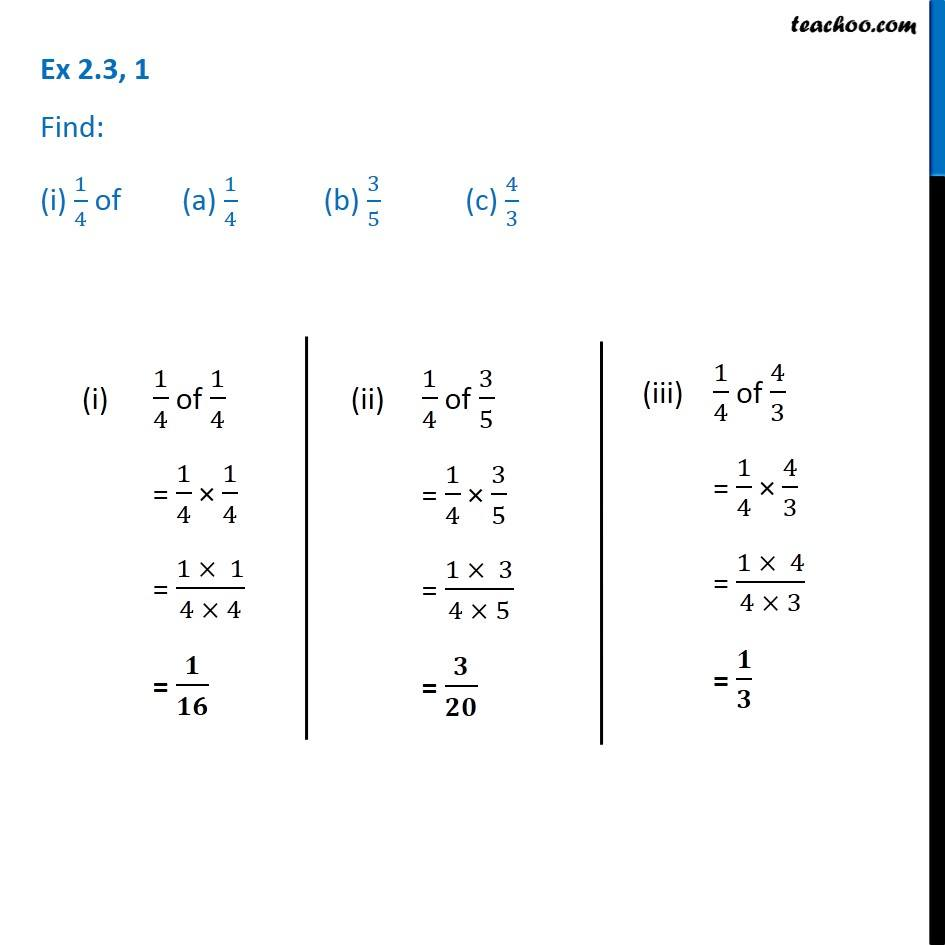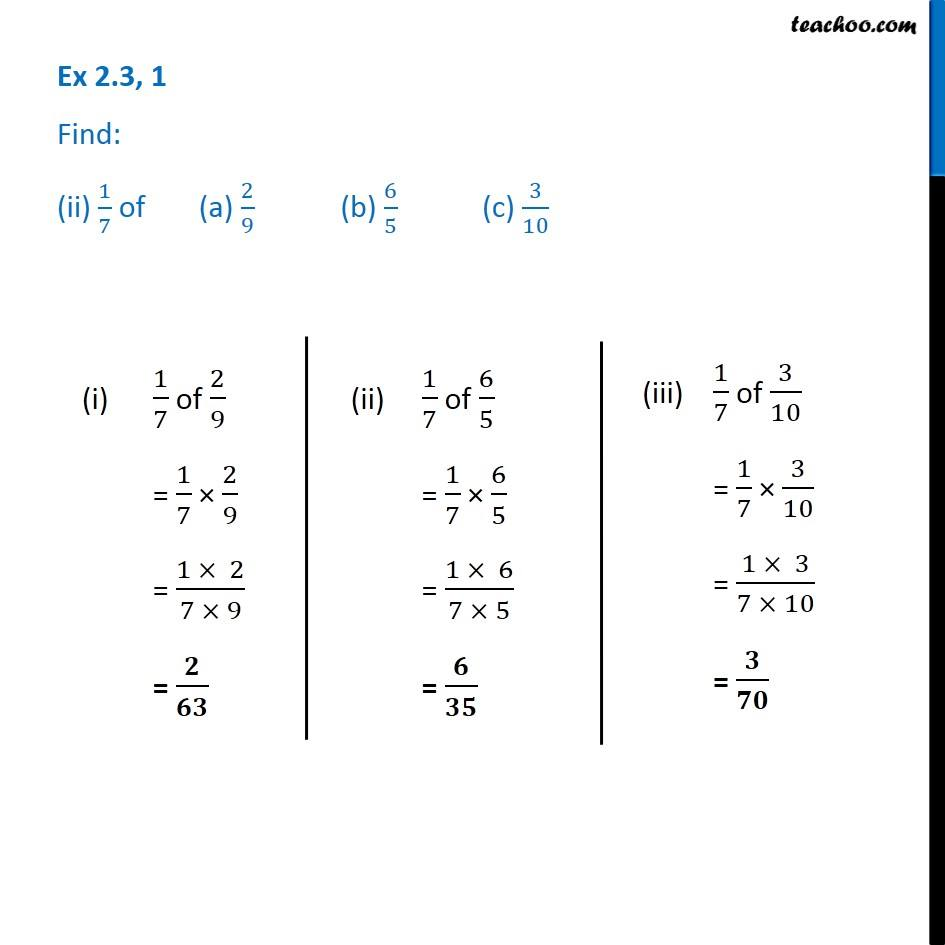Ex 2.2

Chapter 2 Class 7 Fractions and Decimals
Serial order wiseLearn in your speed, with individual attention - Teachoo Maths 1-on-1 Class

### Transcript

Ex 2.2, 1 Find: (i) 1/4 of (a) 1/4 (b) 3/5 (c) 4/3 (i) 1/4 of 1/4 = 1/4 × 1/4 = (1 × 1)/(4 × 4) = 𝟏/𝟏𝟔 (ii) 1/4 of 3/5 = 1/4 × 3/5 = (1 × 3)/(4 × 5) = 𝟑/𝟐𝟎 (iii) 1/4 of 4/3 = 1/4 × 4/3 = (1 × 4)/(4 × 3) = 𝟏/𝟑 Ex 2.2, 1 Find: (ii) 1/7 of (a) 2/9 (b) 6/5 (c) 3/10 (ii) 1/7 of 6/5 = 1/7 × 6/5 = (1 × 6)/(7 × 5) = 𝟔/𝟑𝟓 (iii) 1